Tamil Nadu Board of Secondary EducationSSLC (English Medium) Class 6th

# Tamil Nadu Board Samacheer Kalvi solutions for Class 6th Mathematics Term 1 Answers Guide chapter 6 - Information Processing [Latest edition]

#### Chapters## Chapter 6: Information Processing

Exercise 6.1
Exercise 6.1 [Pages 117 - 119]

### Tamil Nadu Board Samacheer Kalvi solutions for Class 6th Mathematics Term 1 Answers Guide Chapter 6 Information ProcessingExercise 6.1 [Pages 117 - 119]

Exercise 6.1 | Q 1 | Page 117

Suppose, you have two shorts, one is black and the other one is blue; three shirts which are in white, blue and red. You again wish to make different combinations, but you always want to make sure that the shorts and shirt that you wear are of different colours. List and check how many combinations are possible now

Exercise 6.1 | Q 2 | Page 117

You have two red and two blue blocks. How many different towers can you build that are four blocks high using these blocks? List all the possibilities

Exercise 6.1 | Q 3 | Page 117

In the following magic triangle, arrange the numbers from 1 to 6, so that you get the same sum on all its sides.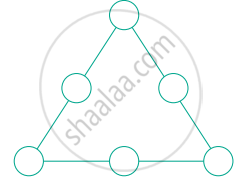Exercise 6.1 | Q 4 | Page 117

Using the numbers from 1 to 9
(i) Can you form a magic triangle?
(ii) How many magic triangles can be formed?
(iii) What are the sums of the sides of the magic triangle?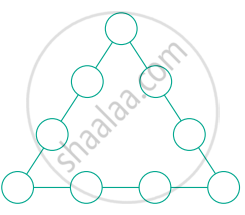Exercise 6.1 | Q 5 | Page 117

Arrange the odd numbers from 1 to 17 without repetition to get a sum of 30 on each side of the magic triangle.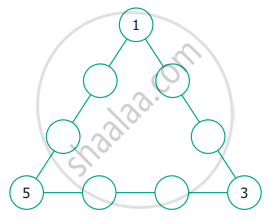Exercise 6.1 | Q 6 | Page 118

Put the numbers 1, 2, 3, 4, 5, 6 and 7 in the circles so that each straight line of three numbers add up to the same total.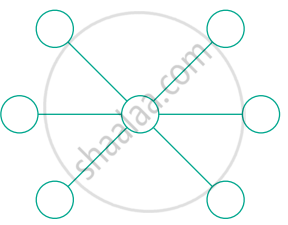Exercise 6.1 | Q 7 | Page 118

Place the number 1 to 12 in the 12 circles so that the sum of the numbers in the six lines of the star is 26. Use each number from 1 to 12 exactly once. Find more possible ways?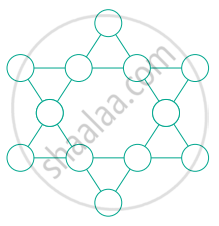Exercise 6.1 | Q 8. (i) | Page 118

How many Triangles are there in the following figure?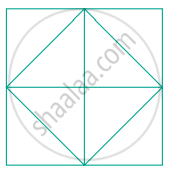Exercise 6.1 | Q 8. (ii) | Page 118

How many Triangles are there in the following figure?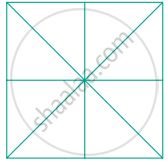Exercise 6.1 | Q 8. (iii) | Page 118

How many Triangles are there in the following figure?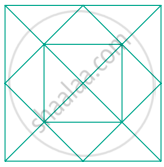Exercise 6.1 | Q 8. (iv) | Page 118

How many Triangles are there in the following figure?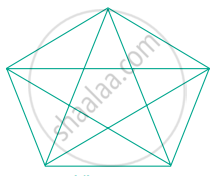Exercise 6.1 | Q 9. (i) | Page 118

Find the number of dots in the tenth figure of the following pattern.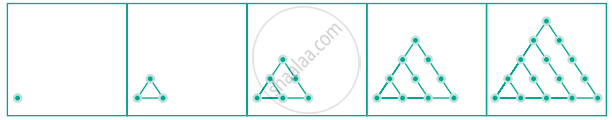Exercise 6.1 | Q 9. (ii) | Page 118

Find the number of dots in the tenth figure of the following pattern.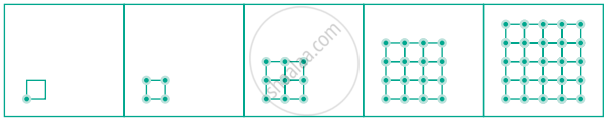Exercise 6.1 | Q 10 | Page 119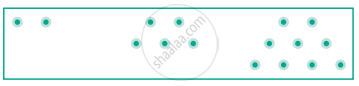(i) Draw the next pattern
(ii) Prepare a table for the number of dots used for each pattern
(iii) Explain the pattern
(iv) Find the number of dots in the 25th pattern

Exercise 6.1 | Q 11. (i) | Page 119

Count the number of squares in the following figure?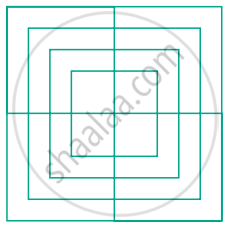Exercise 6.1 | Q 11. (ii) | Page 119

Count the number of squares in the following figure?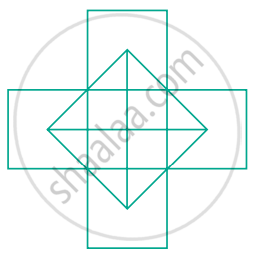Exercise 6.1 | Q 12 | Page 119

How many Circles are there in the following figure?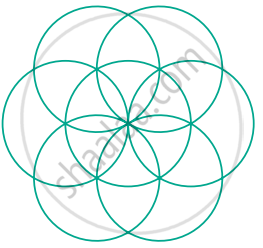Exercise 6.1 | Q 13. (i) | Page 119

Find the minimum number of straight lines used in forming the following figure.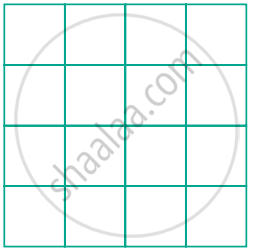Exercise 6.1 | Q 13. (ii) | Page 119

Find the minimum number of straight lines used in forming the following figure.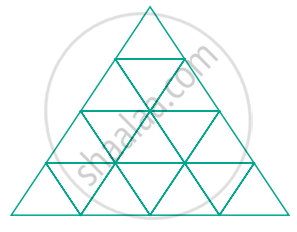## Chapter 6: Information Processing

Exercise 6.1## Tamil Nadu Board Samacheer Kalvi solutions for Class 6th Mathematics Term 1 Answers Guide chapter 6 - Information Processing

Tamil Nadu Board Samacheer Kalvi solutions for Class 6th Mathematics Term 1 Answers Guide chapter 6 (Information Processing) include all questions with solution and detail explanation. This will clear students doubts about any question and improve application skills while preparing for board exams. The detailed, step-by-step solutions will help you understand the concepts better and clear your confusions, if any. Shaalaa.com has the Tamil Nadu Board of Secondary Education Class 6th Mathematics Term 1 Answers Guide solutions in a manner that help students grasp basic concepts better and faster.

Further, we at Shaalaa.com provide such solutions so that students can prepare for written exams. Tamil Nadu Board Samacheer Kalvi textbook solutions can be a core help for self-study and acts as a perfect self-help guidance for students.

Concepts covered in Class 6th Mathematics Term 1 Answers Guide chapter 6 Information Processing are Systematic Listing, Systematic Completion of Lists.

Using Tamil Nadu Board Samacheer Kalvi Class 6th solutions Information Processing exercise by students are an easy way to prepare for the exams, as they involve solutions arranged chapter-wise also page wise. The questions involved in Tamil Nadu Board Samacheer Kalvi Solutions are important questions that can be asked in the final exam. Maximum students of Tamil Nadu Board of Secondary Education Class 6th prefer Tamil Nadu Board Samacheer Kalvi Textbook Solutions to score more in exam.

Get the free view of chapter 6 Information Processing Class 6th extra questions for Class 6th Mathematics Term 1 Answers Guide and can use Shaalaa.com to keep it handy for your exam preparation